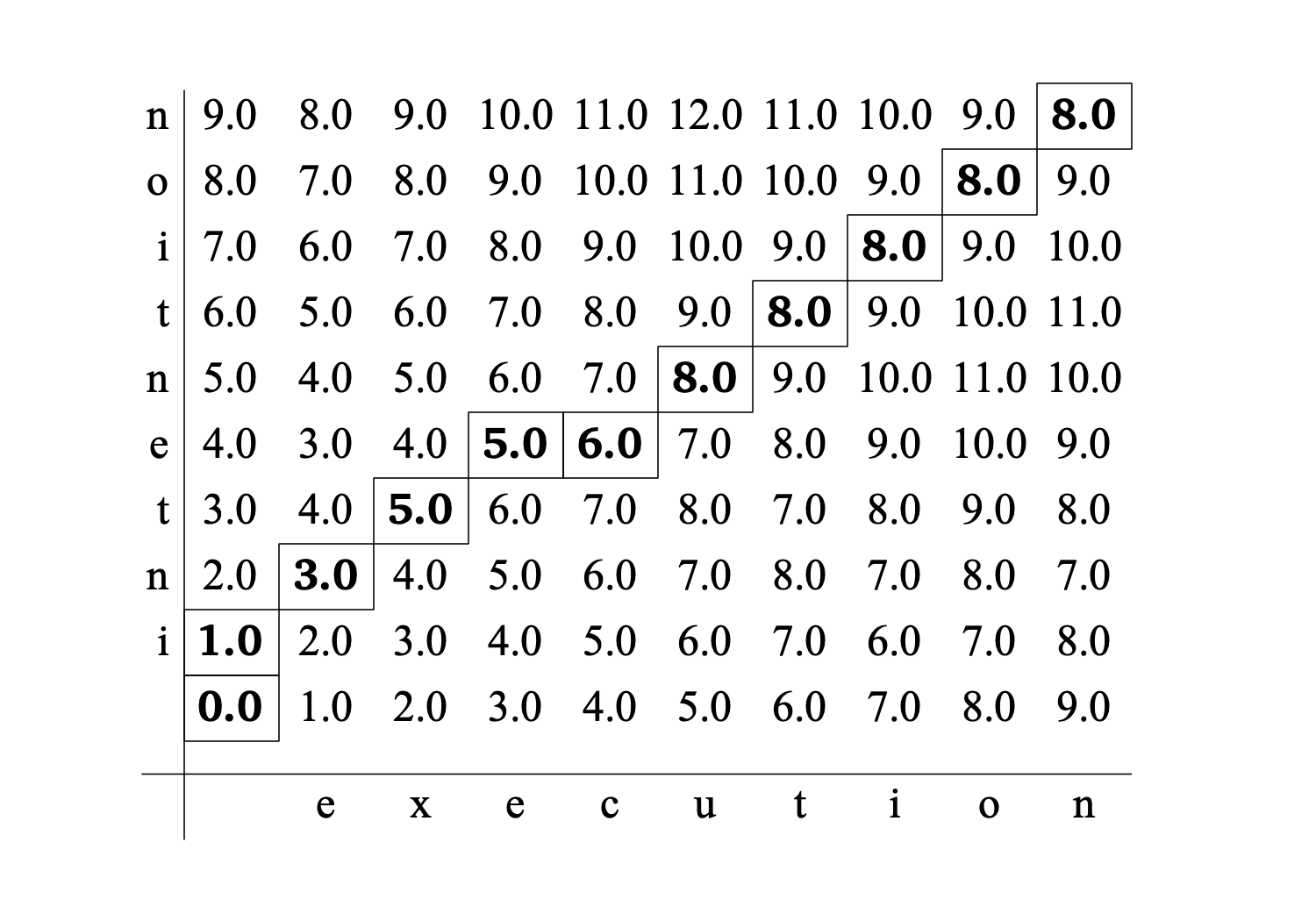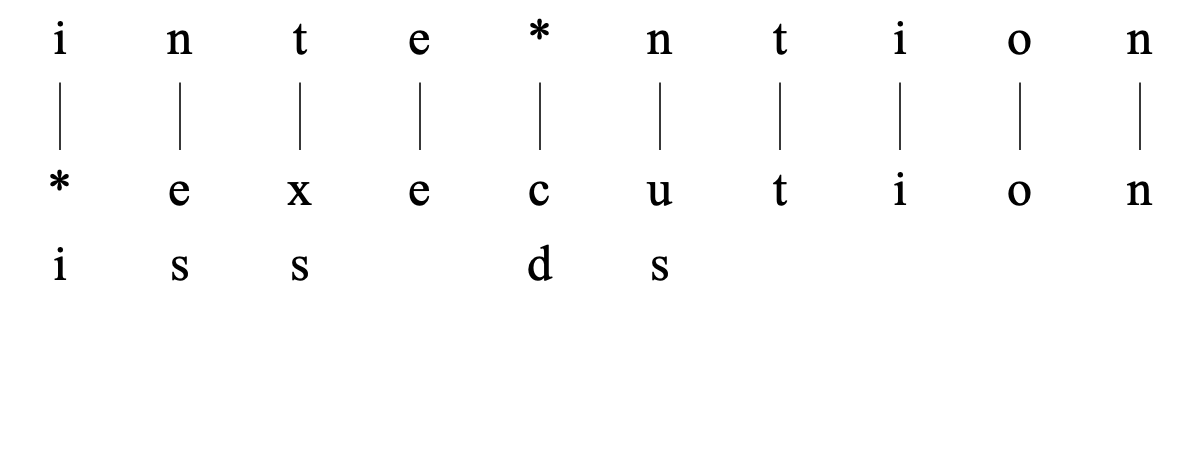EditDistanceTable

One of the types of objects in Praat.

An EditDistanceTable shows the accumulated distances between a target string and a source string. For example, the accumulated distances between the target string "intention" and the source string "execution" can be expressed by the following EditDistanceTable:This figure was created by issuing the following commands:

``` target = Create Strings as characters: "intention"``` ``` source = Create Strings as characters: "execution"``` ``` plusObject: target``` ``` edt = To EditDistanceTable``` ``` Draw: "decimal", 1, 0```

The target string is always displayed vertically while the source string is displayed horizontally and the origin is at the bottom-left corner of the table. Each cell of this table, dist[i, j], contains the accumulated distance between the first i characters of the target and the first j characters of the source. The cells on the path through this table which have the minimum accumulated cost are shown with boxes around them. Below we will explain how this path is calculated.

The local directional steps in this path show which edit operations we have to perform on the source string symbols to obtain the target string symbols. Three edit operations exist: (1) insertion of a target symbol in the source string. This happens each time we take a step in the vertical direction along the path. (2) deletion of a symbol in the source string. This happens each time we take a step in horizontal direction along the path. (3) substitution of a source symbol by a target symbol happens at each diagonal step along the path.

If we trace the path from its start at the origin to its end, we see that it first moves up, indicating the insertion of an "i" symbol in the source string. In the next step which is in the diagonal direction, the "n" target is substituted for the "e" source symbol. Next follows another substitution, "t" for "x". The next diagonal step substitutes "e" for an identical "e". This step is followed by a horizontal step in which the source symbol "c" is deleted. The next diagonal step substitutes an "n" for a "u". The path now continues in the diagonal direction until the end point and only identical substitutions occur in the last part. The following figure shows these operations more explicitly.The value of the accumulated costs in a cell of the table is computed by taking the minimum of the accumulated distances from three possible paths that end in the current cell, i.e. the paths that come from the left, from the diagonal and from below.

``` dist[i,j] = min (dleft, ddiag, dbelow), ```

where

```  dleft = dist[i-1,j] + insertionCost(target[i])``` ```  ddiag = dist[i-1,j-1] + substitutionCost(source[j],target[i])``` ```  dbelow = dist[i,j-1] + deletionCost(source[j])```

Since the calculation is recursive we start at the origin. After calculating the accumulative distances for each cell in the table as based on the algorithm above, the cell at the top-right position will contain the accumulated edit distance. This distance happens to be 8 for the given example. The value 8 results from using the target-indepent value of 1.0 for the insertion cost, the source-independent value of 1.0 for the deletion costs and a constant value of 2.0 for the substitution costs. If target and source symbol happen to be equal no costs are assigned, or, equivalently the substitution costs are zero if target and source symbol match. If you want more control over these costs you can create an EditCostsTable and specify your special costs and then set the new edit costs.

If during the calculations we also keep track of which of the three cells resulted in the local minimum accumulated distance, we can use this directional information to backtrack from the cell at the top-right position to the cell at the bottom-right position and obtain the minimum path.